# How To Build A Go Kart; Part Two: Determining The Center Of Gravity

The go kart looked great. The go kart engine ran great. The 7.5 horsepower engine seemed to just pour forth the power and the go kart had the appearance of being one awesome go kart. That was until the go kart was driven tended to understeer severely. In fact the go kart tended to just go straight versus corner with any predictability. Additionally the go kart was so heavy that it was awkward to drive.

What was the problem with this go kart?

The problem was threefold:

– The Go Kart Was Too Long
– The Center Of Gravity Was Incorrectly Placed
– The Go Kart Was Awkwardly Heavy

From an appearance standpoint the go kart looked good but from a performance standpoint the go kart lacked cornering performance. It was okay in a straight line but was terrible in cornering.

We mentioned that the go kart was too long and that played a role in how the go kart actually cornered as well. The overall ratio between wheelbase and width of the go kart has an important relationship for cornering performance.

Typically the wheelbase of the go kart should be close to what the width of the go kart is or a little bit larger. An excessive amount of wheelbase makes a go kart long and difficult to corner. More what occurs is that you need to have weight out front and it makes the steering clumsy.

Last time in “How To Build A Go Kart Part One” we discussed the general layout of the go kart. Now we are going to discuss how to place the front wheels for optimal center of gravity placement.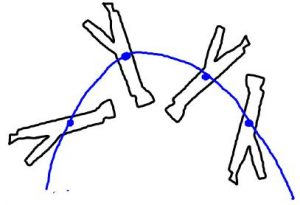Center of Gravity or line of action for the stick

To accomplish this the weight of every component needs to be approximated or measured. If you’re developing a vehicle from scratch

and you want to be precise about the placement of the center of gravity then a precise approximation of the weight of each component is necessary. This may require that you measure the weight of each component prior.

To understand the center of gravity one needs to understand what we mean by that phrase.

The center of gravity is the center of mass of a component. An easy way to understand this is to take a stick and flip it up in the air in a spinning fashion. If you observe the stick as it spins in the air you may notice that one ends sticks out farther than the other end and appears to be lopsided in its rotation. For example flip a hammer in the air and you’ll notice that the steel end is closer to the rotational center then the handle end.

This rotational center is known as the center of gravity or the rotational mass center. In the go kart the center of mass should be at the center point between the wheel base. In other words if your wheelbase is 72 inches then your center of mass will be in the center of 72 inches or at 36 inches from the front wheel or the rear wheel. It would be in the very center between the wheels.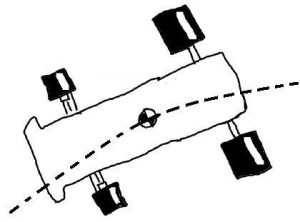Go Kart Center of Gravity

From a practical standpoint the front wheels will have as much weight on them as the rear wheels. This is known as 50-50 weight distribution. To actually achieve this 50-50 weight distribution it can be calculated and then measured afterwards.

Calculating the center of gravity as a whole requires a little understanding of balance equations or teeter totters.

We all understand the big kid on one side of the teeter totter and the small kid on the other side. The big kid in order to not make the small kid fly way up in the air has to go in closer to the fulcrum or pivot point. When the big kid is in the position where the small kid is not flying up in the air this is known as a balance.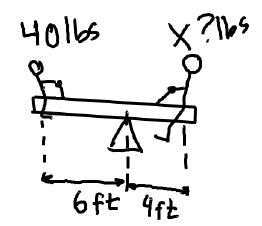Teeter Totter

The balance equations or the center of gravity calculation is very similar to this example. To figure out why the the kids balance out, an understanding of basic force and torque is required.

Torque is essentially a turning force. When something turns in order to resist it from turning you need to apply an opposite turning force. The amount of turning force is related to how large the radius is. For example a large steering wheel is easier to turn up than a smaller steering wheel. Or the amount of force required to turn the wheel is related to the diameter of the wheel. We see this relationship and so we call it torque.

Torque is equal to force times radius.

So the boy on the teeter totter has a distance of 6 feet from the pivot point and the large boy has a distance of 4 feet from the pivot point. We know that the smaller boy weighs 40 pounds how much does the larger boy weigh?

The answers is related to torque. The small boy exerts a torque of 40 pounds times 6 feet which equals 240 foot-pounds. The large boy exerts the same amount of torque (240 foot-pounds) and therefore is balanced on the teeter totter. Knowing this information we can calculate the weight of the larger boy.

The weight of a larger boy equals: 4 feet times unknown weight equals a 240 foot-pounds.

Or in formula layout is as follows:

4ft * X lbs = 240 ft lbs

Solve for X.

X = 240 ft lbs / 4 feet

So X equals 60 pounds. The larger boy weighs 60 pounds. This basic balance equation is what is used to determine the center of gravity for a go kart.

Except now we step up the math a bit. The best way to look at the center of gravity for a go kart is to think about a park bench. A park bench is supported by two supports. When someone sits in the middle how would you determine the weight on each support? That is a basic question you need to ask yourself when you are trying to determine the center of gravity for a go kart.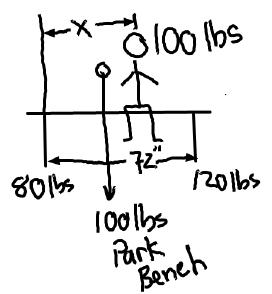Park Bench Problem: Note weight of the man and the bench. Benches weight is on center, whereas the mans weight is off center.

Now instead of having boys sitting on the park bench now you have supports. The center of gravity now is the fulcrum or the pivot point. The trick now is to drive the design so that the center, or the pivot point, is in the middle between the front and the rear wheels.

The best way to drive the center of gravity is to not be flustered by this equation but to understand that parts can move around to adjust the center of gravity. So the radii can change like the big boy moving in on the teeter totter.

So let’s determine the center of gravity for the park bench. We understand that there is 100 pound man sitting on the park bench. We also understand that the park bench itself weighs 100 pounds and is 72 inches wide at the supports. If the weight on one support is 120 pounds and the weight on the other support is 80 pounds where is the person sitting on the park bench?

Well we do a balance equation again. In statics we call this summing the moments. So on a park bench one leg is pushing up with 120 pounds and the man is pushing down with 100 pounds somewhere on the park bench. We can sum the moments about the other leg (the 80 pound leg). This may seem like a roundabout way of calculating, buy is actually quite helpful because it is a math trick. The math trick is designed to simplify the calculation.

We’re going to call the far support leg position “O”. We are going to sum the moments about “O”. To do this we find each corresponding force and multiply it by the radius.

So the equation ends up being the following: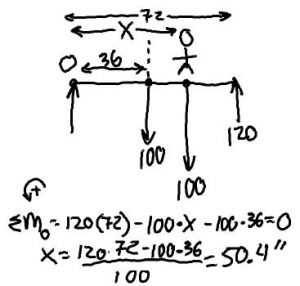Park Bench Calculation

120 * 72 – 100*36-100*X = Zero = 0

Solve for X

X Is the position of the person relative to the far park bench support.

X = 120*72-100*36/100

X = 50.4

The man is sitting 50.4 inches from the far support. We know by this that he is not obviously sitting in the center but is 14.4 inches off center.

Using this same logic we can calculate the center of gravity for the go kart. Basically instead of summing the moments about the far park bench support, we sum the moments about the rear wheel.

The position of the front wheel is the other support for the park bench.

The principle is simple: everything has a center of mass that is a certain distance away from the rear tire. When everything is added together the result is a unified center of gravity reacting as a center of mass for the go kart.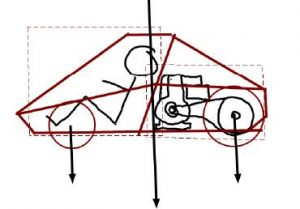Center of Gravity and Front Wheel Placement

Quite honestly we don’t care where the center of gravity is right away, but what we do care about though is how much weight is on the front wheels?

Using the mega formula and powerful spreadsheets we can easily crunch this into a manageable problem. The go kart webpage offers a center of gravity program for free. All you have to do is plug in your weights and your respective distances and it will calculate automatically the resulting weight over the front wheels.

Watch the video below to see how the center of gravity spreadsheet works. Bottom line is that we need to determine what the center of gravity is before we start plowing ahead and making a frame design. We need to make sure that the center of gravity is reasonable and that the go kart is not in danger of oversteer or a severe under-steer.

1. What a fantastic blog! We absolutely love this resource and will be coming back to visit soon.

Keep up the great work.

Pedal Go Karts.org

2. James

Really great work here, although, I can see how my own father’s “father-son projects” turned into engineering projects I couldn’t possibly understand. Do you have any tutorials on how to include my own sons in all of this algebra! lol

• I am coming out with a full blown carriculum that is complete with better videos. So the algebra stuff will become easier to deal with and understand.

3. ashiq

hey, currently i am doing a project of making a chassis for go kart which the running mechanisms is using impact drill. i aldy made it. now the problem that i am facing is about the calculation part. i dont know what calculation i should include for my project report. do u have any suggestion?

• First place to start is the torque level that the drill puts out. You may have to do some simple torque tests or better yet look at the torque rating for the drill. You can then approximate how much HP the drill puts out at the rpm it is spinning. The HP formula is HP = Torque x RPM / 63000. The torque must be in IN-LBs (Inch Pounds). You may have to convert to get to the proper Torque level.

Once you have figured the HP of the drill then you can start doing ratio calcs and figure out the proposed performance curve for your go kart. We talk extensively in our 201, 202 and 203 books about drive systems and how to calculate the forces and performance for your go kart. We also have a free program in our engineering tool kit to put your variables.
Go Kart Engineering Tool Kit
Regards

GKG

4. alan

is the way of determination of centre of gravity correct by using the above method? because it is seem like using 2D approach. how about the weight of engine that contribute to total weight? normally the engine will located at side. so what comment you can say on it?

• Great Question Alan. First yes, you are correct, this is the 2d approach, which is used primarily if you have a go kart that is very symetrical. Once you start moving things from side to side in the vehicle, the line of action shifts. The same type of calculation would be used to figure out the side to side center of gravity as well.

As you stated, what about the center of gravity for say a racing go kart where the engine sits next to the driver. You would instead of figuring out the Teater totter from end to end on the go kart, you would figure it out from side to side. It just gets that much more involved, but important if you indeed need the line of action smack dab in the center of the cart (or there-abouts).

To recap, if you want to find out the CG for two planes, you need to come at it from two directions, namely: lengthwise (as shown in this article) and width wise.

5. STEVE L JANTZEN PE

This is what educators need, real life applications of math directed to something the young person can have an interest in